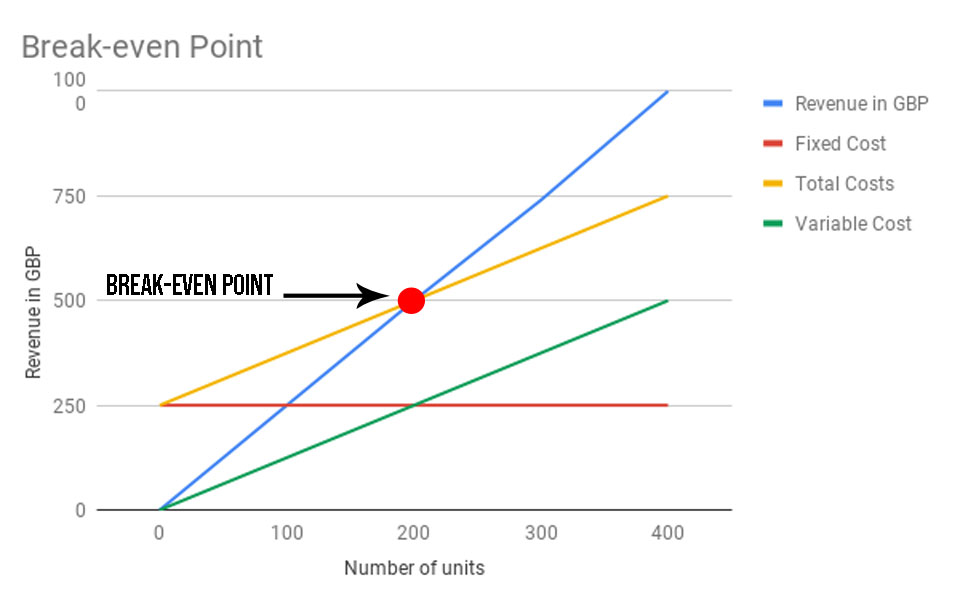Debitoor's accounting dictionary
Break-even point

# Break-even point - What is break-even point?

In accounting, the break-even point is the point at which total revenues equal total costs or expenses, hence, they are “even”.

Keep track of your revenues and expenses with invoicing software. Try Debitoor free for 7 days!

When you reach break-even point, you have no net loss or gain. In other words, you have reached the point where sales revenue exactly covers (and is therefore equal to) total costs, consisting of both fixed costs and variable costs.

## Why break-even is important

For any business, knowing your break-even point is an important tool for long-term planning. It is possible for businesses can have a high turnover ratio, but still be making a loss. Therefore, by knowing your break-even point can help when making decision in areas such as deciding prices, setting sales budgets, and preparing business and operations plans.

By knowing where your break-even point is, you can work out a number of things, such as:

• How many units you need to sell before you start making proft
• How an increase or decrease in price per unit will affect your break-even point
• How your profits will be affected by reducing the price of a unit, or the volume of sales
• How an increase in fixed costs will affect your break-even point

## Break-even point analysis

Break-even point is a key financial analysis tool that is commonly used by many business owners.

In order to calculate the break-even point, you need to know the fixed and variable costs. If you are selling a product, then you need to know these product costs. Once you know these, you can then calculate how many product units you need to sell, or the revenue required in order to cover these costs, and therefore break-even.

To calculate break-even, you can use the following formula:

Break-even point in units = Fixed costs / (Sales price per unit - Variable cost per unit)

Where:

• Fixed costs are those that do not change over time or depending on the productivity of the company.
• Variable costs are those that are affected by fluctuations in production and so changes between given periods.
• Sales price per unit is the selling price of the unit or product.

And just to keep in mind, another name used for Sales price per unit - Variable cost per unit is contribution margin per unit so do not worry if some formulas use this phrase, as it means the same thing.

## Graphically representing the break-even point

This graph shows an example of where break-even point is, in accordance with total costs (made up of both fixed and variable costs).Where number of units is represented on the X axis (horizontal), and revenue in GBP is represented on the Y axis (vertical).

This graphs indicates that break-even point is reached when you have sold 200 units, and received a revenue of 500GB.

Before the break-even point, the area below total costs (yellow line) and above revenue in GBP (blue line) is considered loss. And after the break-even point, the area above the total costs (yellow line) and below revenue in GBP (blue line) is considered profit.

## To sum things up:

• Break -even point: when revenue = total costs (where total costs = variable cost + fixed cost)
• Profit: when revenue > total cost
• Loss: when revenue < total cost